• 介绍了水轮发电机同步电抗的计算，具有参考价值。
• 介绍了如何计算同步电抗，内容具体，具有参考价值。
• 同步发电机组的建模,同步发电机组的建模同步发电机组的建模
• ABB同步发电机低压船用发电机AMG使用手册pdf,ABB同步发电机低压船用发电机AMG使用手册
• LC滤波器虚拟同步发电机模型，2015b matlab，无功PI控制，有功虚拟惯量阻尼控制
• 理想电机假设：1)电机铁心部分的导磁系数为常数;2)电机定子三相绕组完全对称，在空间上互差120度，转子在结构上对本身的直轴和交轴...同步是一种交流电机，主要做发电机用，也可做电动机用，一般用于功率较大，转...
理想电机假设：1)电机铁心部分的导磁系数为常数;2)电机定子三相绕组完全对称，在空间上互差120度，转子在结构上对本身的直轴和交轴完全对称;3)定子电流在空气隙中产生正弦分布的磁势，转子绕组和定子绕组间的互感磁通也在空气隙中按正弦规率分布;4)定子及转子的槽和通风沟不影响定子及转子的电感，即认为电机的定子及转子具有光滑的表面。同步是一种交流电机，主要做发电机用，也可做电动机用，一般用于功率较大，转速不要求调节的生产机械，例如大型水泵，空压机和矿井通风机等。近年由于永磁材料和的发展，微型同步电机得到越来越广泛的应用。的特点之一是稳定运行时的转速n与定子电流的频率f1之间有严格不变的关系，即同步电动机的转速n与旋转磁场的转速n0相同。“同步”之名由此而来。同步发电机是系统中的，它的稳态特性与暂态行为在电力系统中具有支配地位。虽然在电机学中已经学过同步电机，但那时侧重于基本电磁关系，而现在则从系统运行的角度审视发电机组。1.同步发电机的相量图设发电机以滞后功率因数运行，三相同步发电机正常运行时，定子某一相空载电势eq，输出电压或端电压u和输出电流i间的相位关系如图1所示。δ是eq领先u的角度，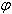称为功角，是功率因数角，即u与i的相位差， eq与q轴(横轴或交轴)重合，d为纵轴或直轴。u和i的d、q分量为：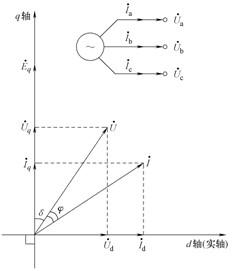图 1 电势电压相量图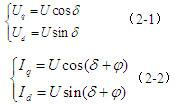电机学课程中已经讨论过，端电压和电流的分量与eq间的关系为：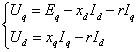(2-3)式中，r为定子每相绕组的电阻，xd为定子纵轴同步电抗，xq为定子横轴同步电抗。其中空载电势eq与转子励磁绕组中的励磁电流成正比，其比例系数可从空载试验中得到。为了便于绘制相量图，令d轴作正实轴，q轴作正虚轴，则各相量可表示为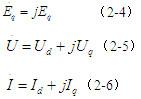所以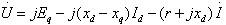(2-7)对于隐极式同步发电机(汽轮发电机)，因气隙均匀，直轴和交轴同步电抗相等(xd=xq)，上式变为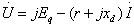(2-8) 此即表示隐极式同步发电机的方程，由此即可作出它的等值电路和相量图，如图2所示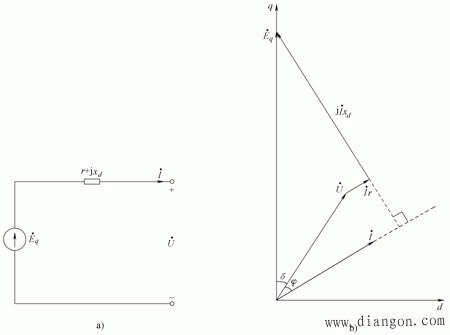(a)等值电路 (b)矢量图图2隐极式同步发电机等值电路和矢量图凸极式同步发电机(水轮发电机)，把电枢反应磁势分解为d轴及q轴两个分量，d轴电枢反应磁势的位置固定在转子d轴上，q轴电枢反应磁势的位置固定在转子q轴上，从而解决了合成磁势遇到的不同气隙宽度的困难。d轴及q轴电枢反应磁势所产生的气隙磁通密度虽不是正弦形(气隙不均匀)，但由于磁路的对称性，其基波轴线仍分别处在d轴及q轴线上，从而可以用叠加定理求取合成电势。因气隙不均匀，直轴和交轴同步电抗不相等，只能用式(2-7)表示，为便于计算，定义了一个与eq同相的虚构电势eq，发电机电压方程为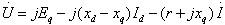(2-9) 定义，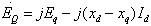则有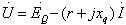(2-10) 式中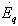相量由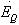和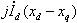两个相量组成，均在q轴上，而由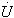及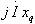求得。凸极式发电机正常运行时的相量图如图2-3(b)所示，在图中利用决定q轴及d轴，即可求得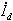，在求得，其等值电路如图2-3(a)所示。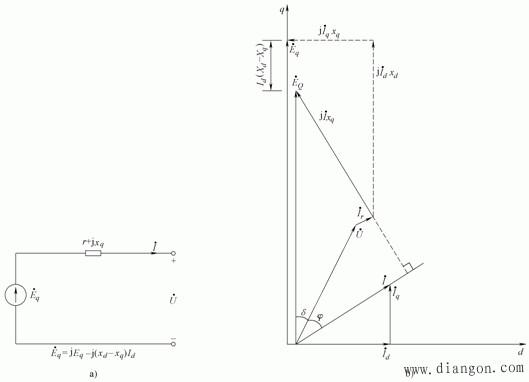(a)等值电路 (b)矢量图图2-3凸极式同步发电机等值电路和矢量图2. 同步发电机的功率特性若取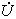为参考向量，领先的角度设为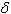，则有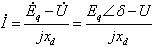(2-11) 隐极式同步发电机输出的电磁功率为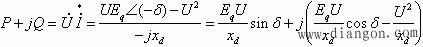(2-12)其中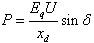(2-13)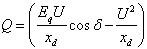(2-14) 式(2-14)就是隐极式发电机的功率与功率角的关系式。其中同步电抗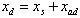，以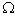为单位，其中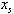为电枢漏抗，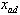为电枢反应电抗。电势与电压取线电势及线电压的有效值，则功率表示为三相功率的有效值。凸极式同步发电机输出的电磁功率为：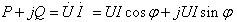(2-15) 其中：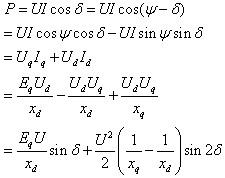(2-16)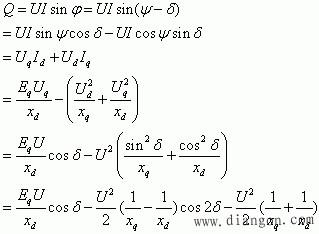(2-17) 式中，其中直轴同步电抗xd=xs+xad，交轴同步电抗xq=xs+xaq，以为单位，其中xs为电枢漏抗，xad为直轴电枢反应电抗，其中xaq为交轴电枢反应电抗。以上各定子回路方程和功率方程就是同步发电机正常运行状态的数学模型。
展开全文• 同步发电机的电磁设计，王拓，肖远，同步发电机在国民生产中有广泛的应用。作为发电机运行是同步电机最主要的运行方式。同步发电机的功率因数可以调节，在不要求调速
• 随着风力发电容量占电网容量的...的逆变器采取基于同步发电机模型的控制策略，将风电场等效为虚拟同步发电机（Virtual Synchronous Generator-VSG）， 使风电场向电网输送的功率平滑，并对大电网体现同步发电机的特性。
• 随着分布式电源渗透率的不断提升，... 首先介绍了同步发电机频率及电压控制的机理，接着介绍并分析了现有的两大类具有典型性的虚拟同步发电机技术方案及其控制原理，最后对虚拟同步发电机技术的发展方向进行了展望。
• 我们公司生产的永磁发电机的优势在于可以做出低转速的永磁发电机，最低转是现在市场上自有我们公司是可以做到的，所以永磁低转速的发电机是相当具备技术含量的， 80kw低速153永磁1585发电机5683 ...
   我们公司生产的永磁发电机的优势在于可以做出低转速的永磁发电机，最低转是现在市场上自有我们公司是可以做到的，所以永磁低转速的发电机是相当具备技术含量的，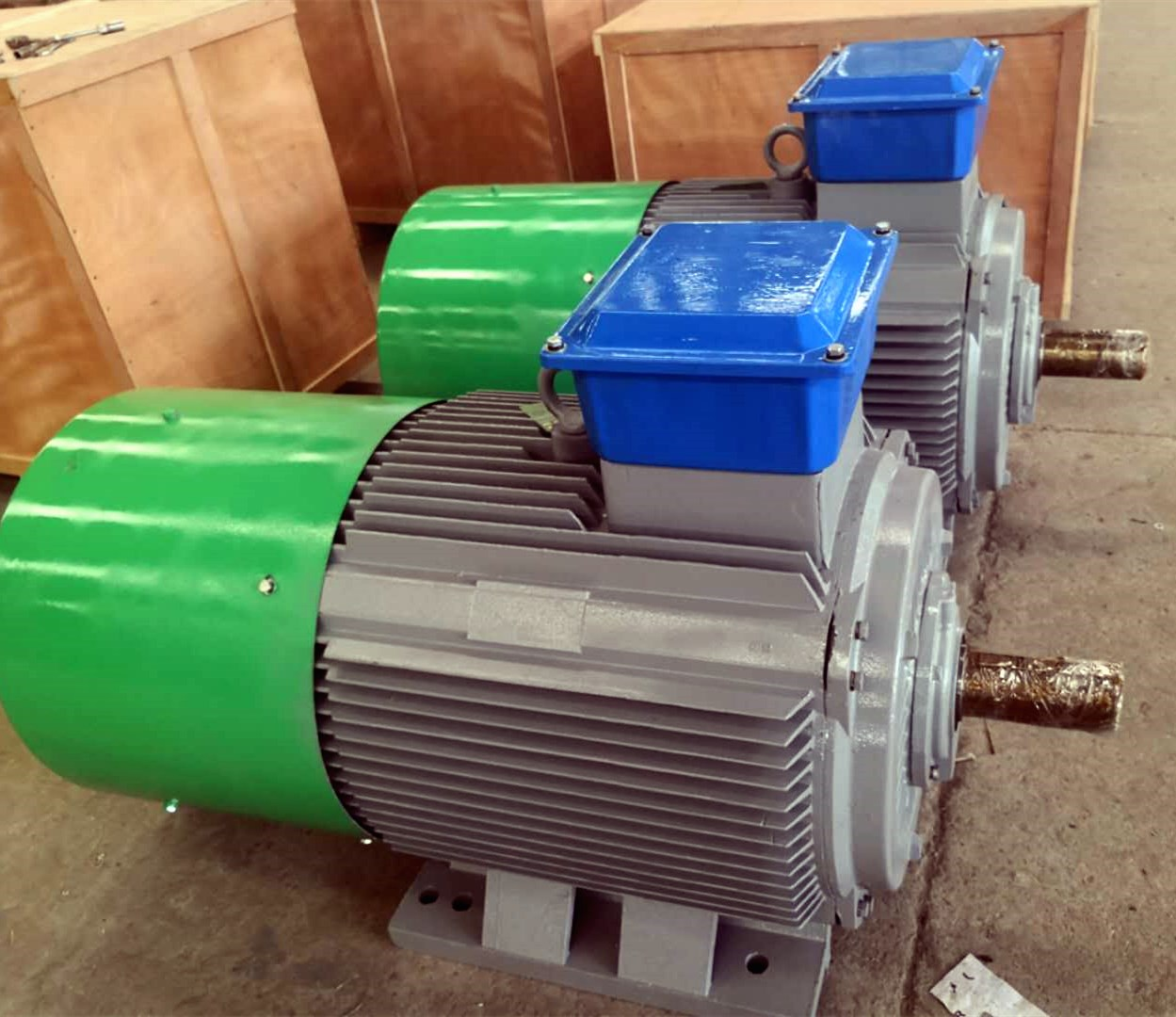80kw低速153永磁1585发电机5683


展开全文• 同步发电机励磁系统的建模与控制-同步发电机励磁系统的建模与控制.rar 摘要：分析了同步发电机交流励磁系统 同步发电机励磁系统的建模与控制.rar 中存在的问题，提出应用斩波技术对其进行...matlab
• 同步发电机励磁原理培训.pdf 介绍了关于同步发电机励磁原理培训的详细说明，提供调节器的技术资料的下载。
• matlab开发-永磁同步发电机。基于永磁同步发电机的风力发电
• simulink仿真文件，一个DC/AC的电路逆变器控制，使用虚拟同步发电机技术（VSG）控制方法，运行顺利，仿真结果波形良好。
• matlab开发-通过与同步发电机协调的风力发电资源自动发电控制。同步发电机协调风力发电资源的自动发电控制
• ABB高压和低压发电机 柴油和天然气同步发电机（英文介绍）pdf,ABB高压和低压发电机 柴油和天然气同步发电机（英文介绍）
• 应用Matlab强大的建模和仿真能力，在Matlab／Simulink中搭建PMSM矢量控制系统的仿真模型，这为PMSM 伺服控制系统的分析与设计提供了有效的手段和工具matlab
• 为提高基于电力电子逆变接口的分布式电源对电力系统保持稳定所需惯性的贡献，设 计了一种基于虚拟同步发电机的新型整体控制策略。此文件包含微电网虚拟同步发电机的仿真步骤，能够对你了解这个领域很有帮助。很实用
• 琢磨了好长时间的异步发电机同步电动机是怎么运行的，终于琢磨出来了，写出来帮我记记。 1、异步电动机与同步发电机原理 这个两个也是最常见的情况，原理不难理解。 异步电动机以鼠笼式的为例：定子通入三相对...


琢磨了好长时间的异步发电机和同步电动机是怎么运行的，终于琢磨出来了，写出来帮我记记。

1、异步电动机与同步发电机原理

这个两个也是最常见的情况，原理不难理解。

异步电动机以鼠笼式的为例：定子通入三相对称电流，气隙中产生以同步速n1旋转的基波磁动势Fa（n1=60f/p，f为电流频率，p为电机极对数），进而建立基波旋转磁场。基波旋转磁场在短路的转子绕组中产生感应电动势，进而在转子中产生感应电流，这个电流与气隙中的旋转磁场相互作用，电磁力便在转子绕组上产生了，转子自然而然地开始旋转。
同步电机：与异步电机不同的是，同步电机转子上有励磁电流或直接装有永磁体，即转子上自发有励磁磁动势Ff产生。用原动机拖动转子至同步速n1（n1=60f/p，f是电网频率，p为电机极对数），那么励磁磁动势Ff会以同步速n1切割定子绕组，在定子绕组中产生频率为f的三相交流电。
2、 异步发电机与同步电动机原理

这个是一个很有意思的问题，其实现方法类似于物极必反的思想，科学归于最后都是哲学。着实很有意思。

异步发电机：
我开始的疑惑在于：既然是发电机，就是让原动机带着转子旋转 。对于鼠笼式异步电机，转子转就是一个铁框框瞎转，而定子侧呢没通电就是一堆线和铁块，这不是对牛弹琴嘛？转子侧跟定子侧哪里来的电磁能量交换？

从下面这幅图入手：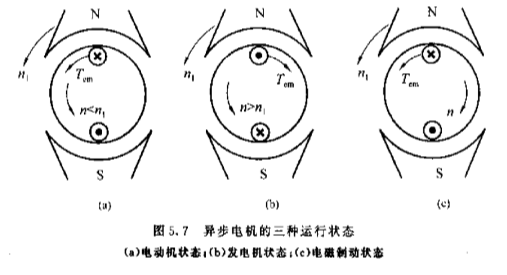其实，异步电机的电动机状态与发电机状态就取决于定转子哪个带着哪个转。

（a）电动机状态：当0<n<n1,即0<s<1时转子中导体以与n相反的方向切割旋转磁场,导体中将产生感应电动势和感应电流。由右手定则,该电流在N极下的方向为向内;由左手定则,该电流与气隙磁场相互作用将产生一个与转子转向同方向的拖动力矩。该力矩能克服负载制动力矩而拖动转子旋转,从轴上输出机械功率。根据功率平衡,该电机一定从电网吸收有功电功率。换句话说，定子产生的旋转磁场对转子产生的拖动力矩使转子实现了转动。

（b）发电机状态：用原动机拖动异步电机,使其转速高于旋转磁场的同步转速,即n>n1，s<0。转子上导体切割旋转磁场的方向与电动机状态时相反,从而导体上感应电动势、电流的方向与电动机状态相反,N极下导体电流方向为向外;电磁转矩的方向与转子转向相反,电磁转矩为制动性质。此时异步电机由转轴从原动机输入机械功率,克服电磁转矩,通过电磁感应由定子向电网输出电功率(因导体中电流方向与电动机状态相反),电机处于发电机状态。换句话说，由于转子转速n大于定子旋转磁场的转速n1，从原来吸收电网功率、克服负载制动力矩转变为输入机械功率、克服电磁转矩，即加大转子转速即可使异步电机实现从电动机到发电机的转变。

实现方法：先使异步电机运行于电动状态，待运行稳定，即转子速达到n后，用原动机拖动转子转速大于n1即可。（理论分析是这样，但工程实际操作肯定不同）

同步电动机
我开始的疑惑在于：同步电机的同步就是励磁磁场Ff转速与旋转磁场Fa转速相同，更深的理解是定转子侧频率相同。异步电机之所以异步是因为如果转子被加速到n1,此时转子导体与旋转磁场同步旋转，它们之间无相对切割，因而导体中无感应电动势,也没有电流,电磁转矩为零。因此异步电机在电动机状态,转速n不可能达到同步转速n1。同步电机于异步电机的区别就在于，同步电机转子上自发建立磁场。

而且对于同步发电机来说，转子是由原动机拖动所以可以达到同步速n1，进而在定子侧产生电网频率的电流。按照异步电动机的思路来思考，同步电动机转子的转速能达不到同步速啊，这不是与同步机的定子冲突了嘛？

这一点我们从同步电动机的转子结构入手：

同步电动机多数在转子上装有类似于异步电机笼型绕组的起动绕组(通称为阻尼绕组),因此,当定子接通电源时,便能产生使转子转动的异步转矩,并不断加速至接近同步速，此时,再加入励磁,就可以用自整步法将电机牵入同步。

换句话说，同步电机的定转子可以类比为两块大磁铁（姑且定义定子为外磁铁，转子为内磁铁），电动机状态或者是发电机状态都可以理解为外磁铁与内磁铁之间的相互带动作用形成的。我们之所以在转子上加上了类似于异步电机笼型绕组的起动绕组，是因为如果在转子静止时就直接通入定子电流，转子是无法转动的。从理解的角度分析，转子一旦通入电流就会产生以速度n1旋转的旋转磁场，即内磁铁静止时，外磁铁突然以速度n1开始高速旋转；内磁铁会因为惯性而无法响应高速旋转的外磁铁。

像那句电影台词，“不是我没动，而是你太慢了。”

所以，先以异步电机的状态启动，待转子达到稍小于同步速n1的转速n稳定后，再加入励磁以同步机状态运行。这时两块磁铁就可以在磁力的相互作用下，共同达到同步速。

3、几个有趣的问题：

什么是同步？什么是异步？
异步的本质是因为异步机必须要有相对运动才会有转矩。

同步机作为发电机时，要考虑到发电频率与电网相同，所以原动机必须以同步速n1旋转；作为电动机，定转子相当于两个磁铁吸引旋转，速度也是相同的。

为什么再说旋转磁场基波Fa以n1旋转时一定要强调基波？而励磁磁场Ff时不需要强调？
对于励磁磁场Ff书上这样写的：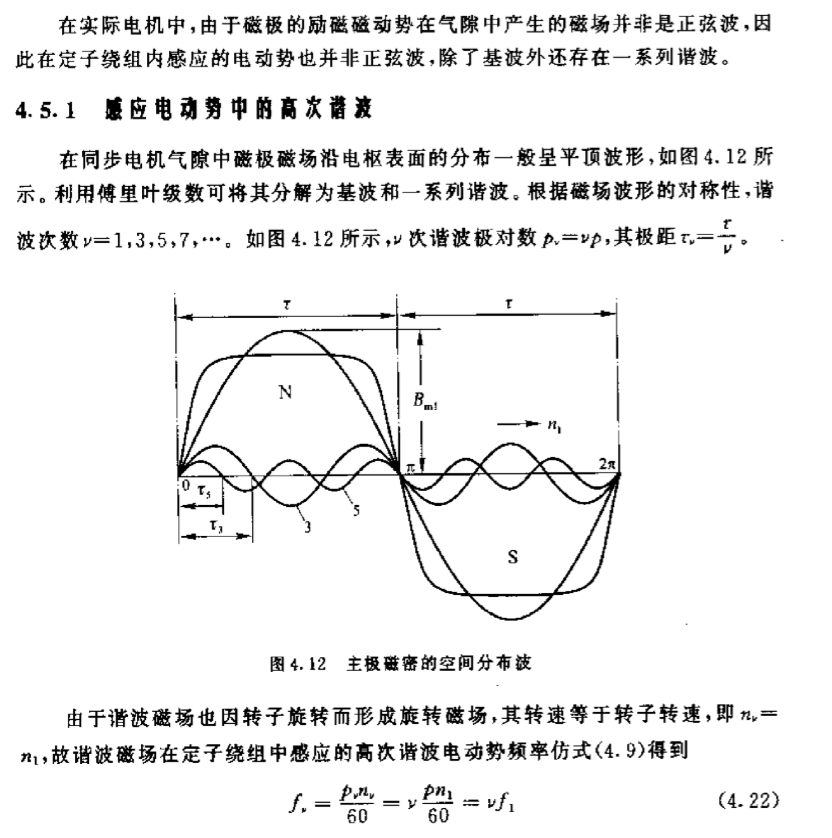由于励磁磁场是“绑在”电机上的，无论基波、谐波转速均为n1；谐波频率为基波的谐波次数倍。

对于旋转磁场磁场Fa书上这样写的：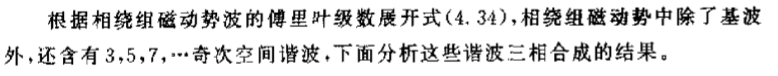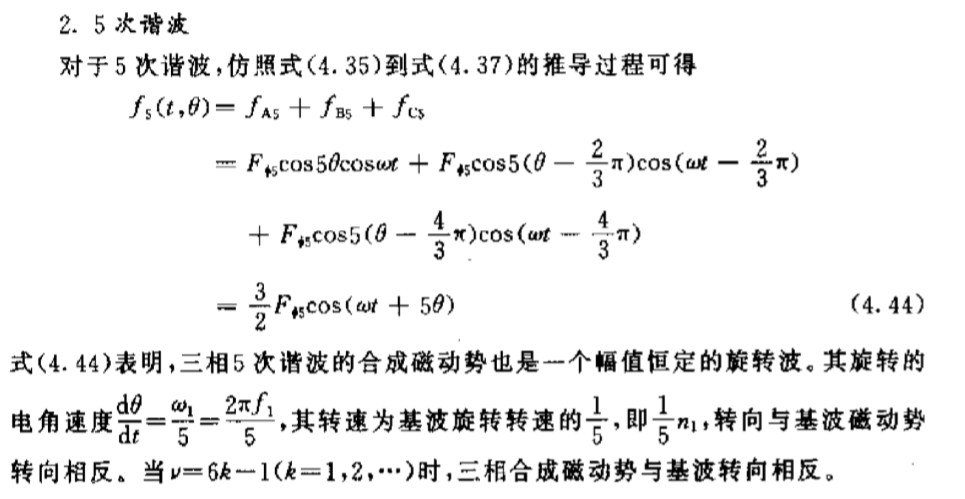即基波、谐波的转速不同，谐波转速为谐波次数分之一；但二者频率相同，均为f。

假如这篇文章对你有帮助，请点个赞鼓励一下我呀！

展开全文• 减少柴油发电机（DG）燃料消耗的解决方案之一是使... 已使用配备有永磁同步发电机（PMSG）的500千瓦柴油GENSET进行了实验分析。 数值和实验结果吻合良好，表明在低电负载下使用旋转式定子用于PMSG可以降低燃油消耗。
• ABB低压同步发电机宣传册pdf,ABB低压同步发电机宣传册
• 有关同步发电机的论文收集 基于变论域模糊PID控制的同步发电机励磁研究 基于模糊自调整PID控制的同步发电机励磁研究 同步发电机模糊PID励磁控制研究 同步发电机模糊PID励磁控制器仿真研究 基于模糊PID控制的同步发电...
• 同步发电机阻尼绕组突然三相短路，各绕组电流编程仿真
• 该实例是对同步发电机进行暂态仿真。例子里面有同步发电机、调速器、励磁系统的详细模型，非常真是的模拟出同步发电机在发生短路情况的，发电机的输出响应。
• 同步发电机转子的转动惯量与运动方程（二） 同步发电机的转子运动方程 由于问题已经特化，因此在（一）部分的计算公式中，转矩、角速度等矢量都共线，此时为了简化计算，如果不做特殊说明，下面的公式均为标量计算。...
同步发电机转子的转动惯量与运动方程（二）  同步发电机的转子运动方程
由于问题已经特化，因此在（一）部分的计算公式中，转矩、角速度等矢量都共线，此时为了简化计算，如果不做特殊说明，下面的公式均为标量计算。若出现矢量、相量，则用加粗的形式给出。
2.1转子运动中的物理量
2.1.1 转子运动中的“速度”
需要指出的是，在同步发电机的应用中，由于极对数p的存在，而角速度ω又指的是电气角速度，转子在进行旋转时又会有一个机械角速度Ω。因此要明确机械角速度Ω、电气角速度ω、定子电流频率f之间的关系：$ω = pΩ$  $f = \frac {np} {60}$ $ω = 2πf$ 式子中，Ω为机械角速度（rad·s-1），ω为电气角速度（rad·s-1），f为定子电流频率（Hz，s-1），p为极对数。依照电力行业标准，我国电力系统的标准电流频率为50 Hz；而同步发电机根据一次能源的不同，极对数p会有不同的选择：例如，对于水轮机而言，以凸极机居多，若采取四对极设计，则其额定转速为nN=750 r/min，对汽轮机而言，以隐极机居多，即只有一对极，则其额定转速为 nN=3000 r/min。
2.1.2 一些会用到的标幺值

物理量（有名值）
标幺值基准值
量纲备注

力矩（转矩）T
$T_B = \frac {S_B} {Ω_0}$
SB：VA（N·m·s−1，kg·m2·s−3）

电气角速度ω
$ω_B = ω_0 = 2πf_0$
ω0为同步角速度，s−1

时间t
$t_B = \frac {1} {ω_0} = \frac {1} {2πf_0}$
ω0为同步角速度，s−1

2.1.3 转动惯量与惯性时间常数
发电机组惯性时间常数TJ是指发电机的转轴上加额定转矩TN后，转子从停顿状态加速到额定转速 所需要的时间。直观来讲，如果发电机组惯性时间常数TJ越小，说明转子越容易加速。在标幺值系统中，转动惯量需要转换成惯性时间常数（单位：s）。
在不考虑额外阻尼作用的情况下，同步发电机转子的机械角加速度与作用在其旋转轴上的不平衡转矩应满足：$J \vec α = J \frac {d \vec Ω} {d t} = Δ \vec T = \vec T_T - \vec T_E$ 类似于平移运动中的牛顿第二定律： $m \vec a = m \frac {d \vec v} {d t} = \vec F$ 其中，α为转子机械角加速度（rad·s-2）；Ω为转子机械角速度（rad·s-1）；J为转子的转动惯量（kg·m2）；ΔT为作用在转子旋转轴上的不平衡转矩（N·m kg·m2·s−2），TT为原动机机械转矩（相当于驱动力），TE为发电机电磁转矩（相当于负荷带来的阻力）；t为时间（s）。上述物理量除了J与t之外，都是共线的矢量，因此，在接下来的计算中，可以仅靠其数值进行计算，而使用正负区分方向。
当转子以额定机械角速度Ω0（同步转速）旋转时，其旋转动能Wk（单位：J）为： $W_k = \frac {1} {2} J Ω^2$ $J = \frac {2 W_k} {Ω_0^2}$ $J \frac {d Ω} {d t} = ΔT$ 因此有： $\frac {2 W_k} {Ω_0^2} · \frac {d Ω} {d t} = ΔT$ 转换成标幺值下的表述（标幺值的右下角会加*号）： $\frac { \frac {2 W_k} {Ω_0^2} } { \frac {S_B} {Ω_0}} · \frac {d Ω} {d t} = \frac {2 W_k} {S_B · Ω_0} · \frac {d Ω} {d t} = ΔT_* = T_T* - T_E*$ 同时应满足 $Ω = \frac {ω} {p}$ $Ω_0 = \frac {ω_0} {p_0}$ 其中，ω0 = ωN 均为同步电气角速度。因此，可以归纳出： $\frac {2 W_k} {S_B · ω_0} · \frac {d ω} {d t} = \frac {T_J} {ω_0} · \frac {d ω} {d t} = ΔT_*$ $T_J = \frac {2 W_k} {S_B} = \frac {J Ω_0^2} {S_B} = \frac {J ω_0^2} {S_B · p^2}$ 其中，TJ为发电机组的惯性时间常数（s）。需要注意的是，有些外文文献会使用H来表示惯性时间常数： $2H = T_J$
2.1.4 功角的定义
发电机转子的q轴以电气角速度ω旋转（稳态情况下的发电机空载电动势Eq位于q轴的方向上），端电压U以同步电气角速度ω0旋转。
def. 1 功角
定义功角δ为，发电机转子的q轴与端电压U之间的夹角。
由于q轴（包括Eq）与端电压U在空间中都是旋转的，但是在额定工作状态下，ω=ω0，因此，它们之间的空间位置可以保持相对静止，因此此时功角δ也保持不变。一旦发生扰动（不论扰动的大小），导致ω不等于ω0，此时，δ也会随时间变化： $\frac {d δ} {d t} = ω - ω_0$ $\frac {d^2 δ} {d t^2} = \frac {d ω} {d t}$
2.2 同步发电机的转子运动方程
为了简化分析，接下来的方程都是建立在极对数p为1的基础上的。此时有ω=Ω。因此，在不考虑阻尼的情况下，纯有名值形式下的转子运行方程（摇摆方程）为： $\frac {d δ} {d t} = ω - ω_0$ $J \frac {d ω} {d t} = T_T - T_E = \frac {P_T} {ω} - \frac {P_E} {ω}$ 或者 $\frac {d δ} {d t} = ω - ω_0$ $J ω \frac {d ω} {d t} = P_T - P_E$
2.2.1 运动方程中的第一项标幺化
$\frac {d δ} {d t} = (ω_* - 1) · ω_0$
2.2.2 运动方程中的第二项标幺化
转矩与转速之积为该转矩的功率，且 $\frac {Ω} {Ω_B} = \frac {ω} {ω_B} = ω_*$ 因此在标幺值下，有： $P_T* = ω_* · T_T*$ $P_E* = ω_* · T_E*$ 因此针对运动方程中的第二项： $J \frac {d ω} {d t} = T_T - T_E = \frac {P_T} {ω} - \frac {P_E} {ω}$ 可以按如下形式化简： $\frac {J} {\frac {S_B} {ω_0}} · \frac {d ω} {d t} = \frac {T_T} {\frac {S_B} {ω_0}} - \frac {T_E} {\frac {S_B} {ω_0}} = \frac {P_T} {ω · \frac {S_B} {ω_0}} - \frac {P_E} {ω · \frac {S_B} {ω_0}}$ $\frac {J ω_0^2} {S_B} · \frac {d ω_*} {d t} = T_T* - T_E* = \frac {P_T*} {ω_*} - \frac {P_E*} {ω_*}$ 同时，在标幺值下，一般认为电气角速度ω* 变化不大，一般取ω * = 1 。因此，可以进一步进行简化：$T_J \frac {d ω_*} {d t} = T_T* - T_E* \approx P_T* - P_E*$ 因此，进一步推导出： $T_J \frac {d ω_*} {d t} = P_T* - P_E*$
在标幺值下，可以更加方便地讨论阻尼带来的影响：转子在旋转中受到的风阻、转轴与轴承之间的摩擦力。这些摩擦力对转子产生阻尼转矩，在标幺值下，近似认为该转矩的大小正比于电气角速度ω*，比例系数称为风阻系数D（显然，D没有量纲）。
因此，考虑阻尼效果的运动方程中的第二项的表达为： $T_J \frac {d ω_*} {d t} = P_T* - P_E* - D(ω_* - 1)$
2.2.3 最常用转子运动方程形式
综合2.2.1与2.2.2，就可以得到最常用的转子运动方程的标幺形式： $\frac {d δ} {d t} = (ω_* - 1)ω_0$ $T_J \frac {d ω_*} {d t} = P_T* - P_E* - D(ω_* - 1)$ 再省略表征标幺值的下标“*”，就得到了教材上最常见的形式： $\frac {d δ} {d t} = (ω - 1)ω_0$ $T_J \frac {d ω} {d t} = P_T - P_E - D(ω - 1)$
虽然看起来这个方程组是线性的，但我们在这里并没有给出PE的完整表达，它的存在就已经决定了方程组的非线性，并在接下来的分析里给我们带来无穷无尽的麻烦：这一组方程将是以小干扰法分析静态稳定的最核心方程。
2.2.4 不同形式的转子运动方程
根据电气角速度ω、时间t是有名值还是标幺值，标幺值下的转子运动方程有多种表达：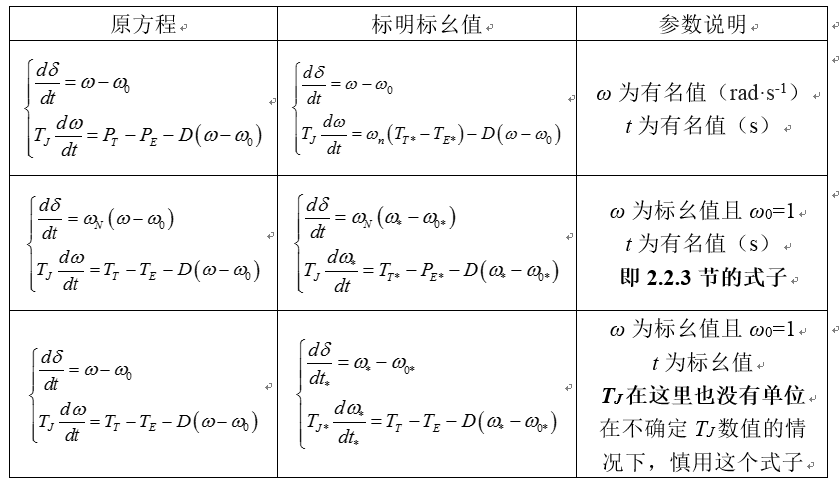不管怎样，在使用转子运动方程时，一定要做的就是通过判断各个物理量的量纲，以确定各个物理量是标幺值还是有名值，尤其是确定时间t是标幺值还是有名值： $t_* = t \times t_B = 100πt$ 如果时间t使用错误，会导致我们在用错误的时间尺度研究问题，自然得不到正确的结果。
另外，在不同的文献中，同步电气角速度的变量形式各不相同，但是值都是一样的：$ω_0 = ω_N = ω_B = 2πf_N$ 量纲为：rad·s-1。
通过判断电气角速度ω的量纲，可以反推出余下所有物理量的量纲，进而判断各物理量是标幺值还是有名值。
第一部分：基本物理概念
https://blog.csdn.net/qq_38642223/article/details/89289002
参考文献
	李光琦. 电力系统暂态分析（第三版）.
	王锡凡. 现代电力系统分析.
	https://en.wikipedia.org/wiki/Swing_Equation .


展开全文转动惯量 转子运动方程
• 同步发电机励磁系统建模导则 同步发电机励磁系统建模导则 同步发电机励磁系统建模导则...